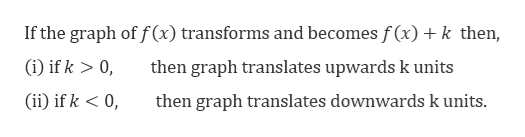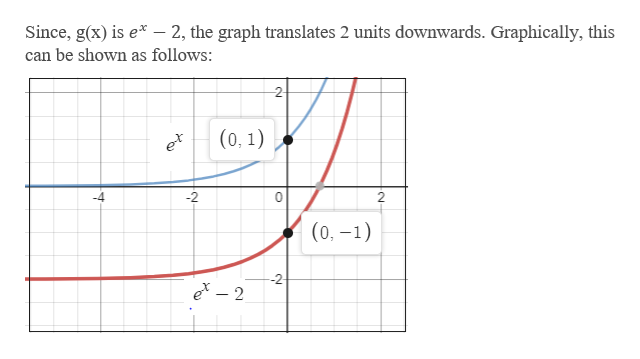# 2.5.15Describe verbally the transformation(s) that can be used to obtain the graph of g from the graph of f.g(x) = ex- 2; f(x) = exSelect the correct choice below and, if necessary, fill in the answer box(es) within your choice.(Type integers or simplified fractions.)O A. The graph of g is the graph of f reflected in the y-axis.O B. The graph of g is the graph of f shiftedunit(s) down.C. The graph of g is the graph of f shiftedunit(s) to the left.unit(s) to the left and stretched horizontally by a factor ofOD. The graph of g is the graph of f shiftedE. The graph of g is the graph of f shiftedunit(s) up.OF. The graph of g is the graph of f shiftedunit(s) down and stretched horizontally by a factor ofO G. The graph ofg is the graph of f reflected in the x-axis.OH. The graph of g is the graph of f shiftedunit(s) up and stretched horizontally by a factor ofI.The graph of g is the graph of f shiftedunit(s) to the right.O J. The graph of g is the graph of f shiftedunit(s) to the right and stretched horizontally by a factor of

Question
17 viewshelp_outlineImage Transcriptionclose2.5.15 Describe verbally the transformation(s) that can be used to obtain the graph of g from the graph of f. g(x) = ex- 2; f(x) = ex Select the correct choice below and, if necessary, fill in the answer box(es) within your choice. (Type integers or simplified fractions.) O A. The graph of g is the graph of f reflected in the y-axis. O B. The graph of g is the graph of f shifted unit(s) down. C. The graph of g is the graph of f shifted unit(s) to the left. unit(s) to the left and stretched horizontally by a factor of OD. The graph of g is the graph of f shifted E. The graph of g is the graph of f shifted unit(s) up. OF. The graph of g is the graph of f shifted unit(s) down and stretched horizontally by a factor of O G. The graph of g is the graph of f reflected in the x-axis. OH. The graph of g is the graph of f shifted unit(s) up and stretched horizontally by a factor of I. The graph of g is the graph of f shifted unit(s) to the right. O J. The graph of g is the graph of f shifted unit(s) to the right and stretched horizontally by a factor of fullscreen
check_circle

Step 1

Given

Step 2

We know thathelp_outlineImage TranscriptioncloseIf the graph of f(x) transforms and becomes f(x)k then, (i if k> 0, then graph translates upwards k units (ii if k < 0 then graph translates downwards k units. fullscreen
Step 3

Now, ...help_outlineImage TranscriptioncloseSince, g(x) is e- 2, the graph translates 2 units downwards. Graphically, this can be shown as follows: (0, 1) (0,-1) 2 ex - 2 fullscreen

### Want to see the full answer?

See Solution

#### Want to see this answer and more?

Solutions are written by subject experts who are available 24/7. Questions are typically answered within 1 hour.*

See Solution
*Response times may vary by subject and question.
Tagged in

### Other